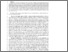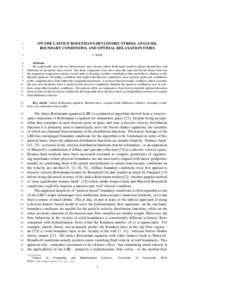# On the lattice Boltzmann deviatoric stress: analysis, boundary conditions, and optimal relaxation times

(2020) On the lattice Boltzmann deviatoric stress: analysis, boundary conditions, and optimal relaxation times. SIAM Journal on Scientific Computing, 42 (2). B397-B424. ISSN 1064-8275 (Print), 1095-7197 (Online) (doi:https://doi.org/10.1137/19M1244846)Preview
PDF (Author's Accepted Manuscript)
26766 REIS_On_The_Lattice_Boltzmann_Deviatoric_Stress_(AAM)_2020.pdf - Accepted Version

## Abstract

We analytically solve the {two dimensional, nine-velocity,} lattice Boltzmann model in planar channel flow and determine its deviatoric stress tensor. The shear component of its stress takes the expected Navier-Stokes form but the tangential component contains second order in Knudsen number contributions that one finds in solutions to the Burnett equations. Boundary conditions that neglect this Burnett contribution cause spurious grid-scale oscillations in the computed stress field within the computational domain. A moment-based boundary condition which considers the non--zero deviatoric stress is analysed and shown to completely eliminate the spurious oscillations seen in solutions using other boundary conditions. The analysis offers an explanation of previously reported optimal relaxation times in terms of the recurrence relation for the tangential stress and gives them an interpretation in terms of compact finite difference schemes.

Item Type: Article Lattice Boltzmann equation, Burnett stress, compact finite difference schemes, boundary conditions, two relaxation time models Q Science > QA Mathematics Faculty of Engineering & Science > School of Computing & Mathematical Sciences (CMS)Faculty of Engineering & Science Publisher 04 Mar 2022 13:06 http://gala.gre.ac.uk/id/eprint/26766View Item